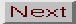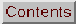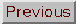3.4 Physical Basis

The physical processes behind the pulsation are relatively easy to understand, although they are by no means trivial to model. The interested reader is directed to Cox (1980, Chapter 10) for a full discussion. Normally, the compression of an element of gas will result in an increased rate of energy loss. However, near the boundary of an ionization zone of an abundant element, the gas can absorb heat when most compressed and release it after the instant of maximum density. If this interaction occurs at a resonant radius in the interior of a star, pulsation will ensue.

In Cepheid variables, the He+ <=> He++ zone is believed to be responsible for most of the driving. However, the H <=> H+ zone does have an influence on the form and phasing of the light and velocity curves. The regularity of Cepheid pulsation is astonishing, corresponding to oscillator Q's of 109-1010 in most cases. As clocks, Cepheids are as good as the rotation rate of the earth. The repeatability of Cepheids is a significant asset allowing re-examination at a later date with only minimum calibration time.

The usefulness of Cepheids rests on the obvious correlation of period with luminosity. How does this arise? Every star has a characteristic oscillation period, but pulsation in stars outside the instability strip is strongly damped. The strong period-luminosity correlation arises because the temperature width of the instability strip is so narrow (due, in turn, to the need for the partial ionization zone to be at an appropriate depth in the atmosphere). If the temperature width of the strip were much larger, the period-luminosity relationship would be much less apparent, but a period-luminosity-color (P-L-C) relationship would retain its precision.

This interplay of parameters can be better understood by the following argument. The dependence of the period, P, on the stellar mass, M, and radius, R, follows from Kepler's third law where: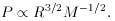(1)

The luminosity of the star, L, is correlated with its mass due to the strong density and temperature dependences of the energy generation equations: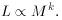(2)

However, luminosity is also determined by the stellar radius and effective temperature, T, by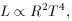(3)

so we can eliminate mass as a variable and the period is determined from two parameters: luminosity and temperature. The corresponding observational quantities are magnitude and color index.

The period-luminosity-color (P-L-C) relation is an equation for a plane. Cepheid variables are found only in the swath of the plane where stars are pulsationally unstable (and where real stars are actually found due to evolution!). Projections of this plane along each axis result in 1) the color-magnitude diagram, 2) the period-color diagram, and 3) the period-luminosity diagram. This is illustrated in Figure 3, where the data on galactic calibrating Cepheids is taken from Table 2 of Feast and Walker (1987). Note that changing the boundaries of the allowed region of instability in the plane does nothing to reduce the quality of the P-L-C fit, but will change the widths of the distributions in all the projected planes. Incomplete filling of the instability strip by evolutionary tracks can therefore affect distance determinations using the P-L relation, but will not affect distances derived from a P-L-C relation. (The reader should note the similarities between the P-L-C plane and the so-called 'fundamental plane' of the Dn -relation.)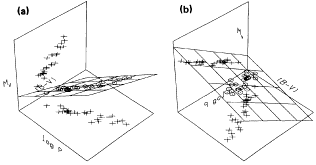Figure 3. Three-dimensional representations of the P-L-C relation given in the text with the points from Table 2 of Feast and Walker (1987) plotted. In (a) the projections onto the color-magnitude and period-color diagram are shown. The viewpoint in (b) has been chosen to highlight the period-luminosity and period-color projections.

Recent work on Galactic and LMC Cepheids by Fernie (1990) and Mateo et al. (1990) has demonstrated that the instability strip does not seem to have a constant width in color. Rather, the instability strip is better described by a wedge in the color-magnitude diagram opening at (B - V) = 0.4, MV = -2 mag with blue and red edges proceeding toward (B - V) = 0.6, MV = -5 mag, and (B - V) = 1.1, MV = -5 mag, respectively. This is most likely due to the filling of the instability region by evolution - the existence of pulsatingScuti stars and white dwarfs is evidence that the instability strip exists at lower luminosities.

The values of the coefficients of the P-L-C are still a topic of active discussion (especially with regard to metallicity dependence). However, a representative P-L-C relation from Feast and Walker (1987) is: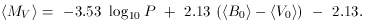(4)

The bracket notation indicates flux-weighting and the zero subscript indicates a dereddened magnitude. Similarly, a representative P-L relation from the same source is: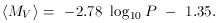(5)